## RS Aggarwal Class 8 Solutions Chapter 4 Cubes and Cube Roots Ex 4A

These Solutions are part of RS Aggarwal Solutions Class 8. Here we have given RS Aggarwal Solutions Class 8 Chapter 4 Cubes and Cube Roots Ex 4A.

Other Exercises

Question 1.
Solution:
(i) (8)³ = 8 x 8 x 8 = 512
(ii) (15)³ = 15 x 15 x 15 = 3375
(iii) (21)³ = 21 x 21 x 21 = 9261
(iv) (60)³ = 60 x 60 x 60 = 216000 Ans.

Question 2.
Solution:
(i)(1.2)³= 1.2 x 1.2 x 1.2 = 1.728
(ii) (3.5)³ = 3.5 x 3.5 x 3.5 = 42.875
(iii) (0.8)³ = 0.8 x 0.8 x 0.8 = 0.512
(iv) (0.05)³ = 0.05 x 0.05 x 0.05 = 0.000125

Question 3.
Solution: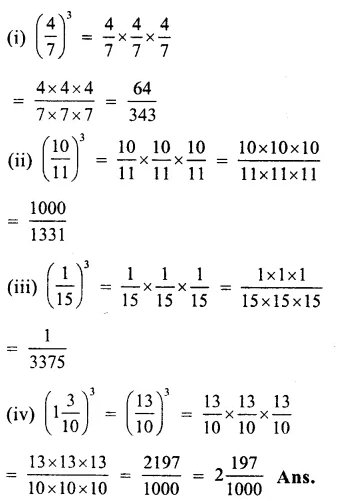Question 4.
Solution:
(i) 125
= $$\overline { 5\times 5\times 5 } ={ \left( 5 \right) }^{ 3 }$$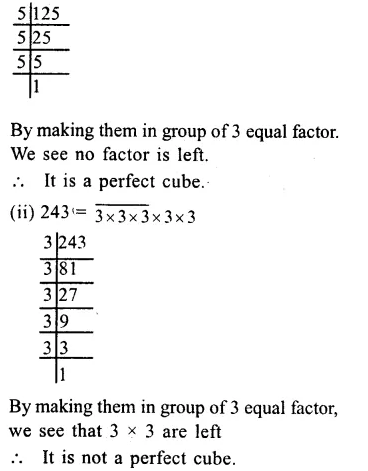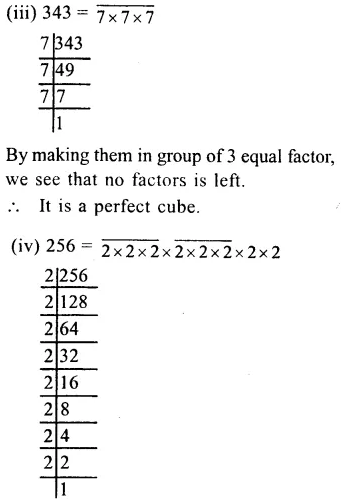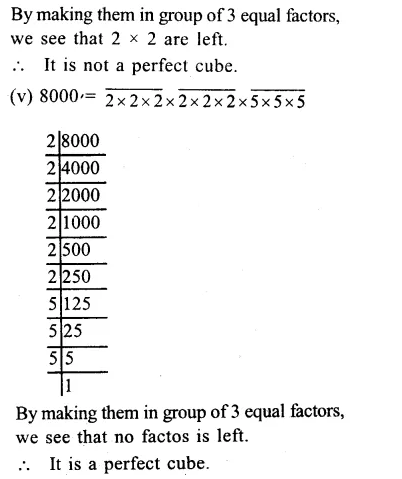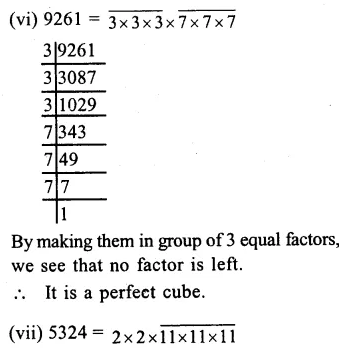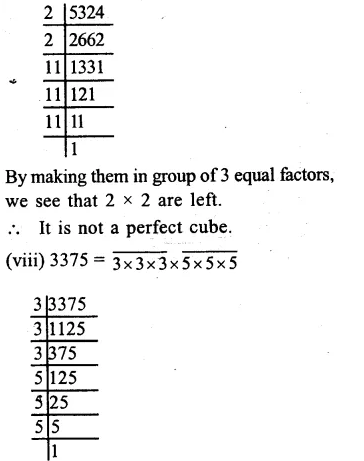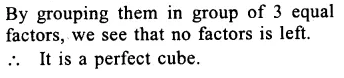Question 5.
Solution:
We know that cube of an even number is also even.
216, 1000 and 512 are the cubes of even numbers as these are all even numbers. Ans.

Question 6.
Solution:
We know that cube of an odd number is also odd.
125, 343 and 9261 are the cubes of odd natural numbers as these are also odd numbers. Ans.

Question 7.
Solution:
Factorising 1323 into prime factors,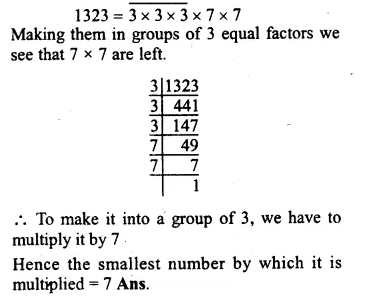Question 8.
Solution:
Factorising 2560 into prime factors.Making them in groups of 3 equal factors, we are left 5
To make it into a group of 3, we have to multiply it by 5 x 5 i.e. by 25.
Hence, the smallest number by which it is multiplied = 25 Ans.

Question 9.
Solution:
Factorising 1600 into prime factors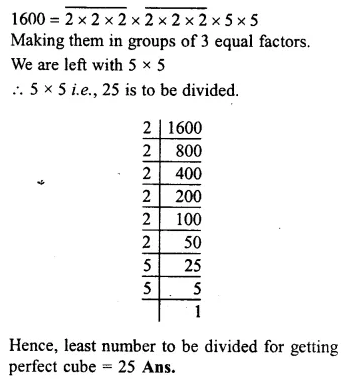Question 10.
Solution:
Factorising 8788 into prime factorsMaking them in groups of 3 equal factors, we are left with 2 x 2
2 x 2 i.e. 4 is to be divided.
Hence least number to be divided for getting perfect cube = 4 Ans.

Hope given RS Aggarwal Solutions Class 8 Chapter 4 Cubes and Cube Roots Ex 4A are helpful to complete your math homework.

If you have any doubts, please comment below. Learn Insta try to provide online math tutoring for you.